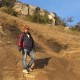Ask Your Question

# Multi cascade detector. LBP

Hello. How to create multi cascade detector with one feature pool (shared features)? I have many detecotrs (LBP) different objects or veiws of object, but they uses own features. Second, I not understand what mean this LBP features:  x=0; y=1; width=2; height=4; In theory LBP is binary histogram. I have one idea that x y is offset and width height is sizes of one of 8 rectangles. (In theory there is squres whith sizes of all pateern 3 6 9 and etc). How can i interpret LBP features in OpenCV?

edit retag close merge delete

## 2 answers

Sort by » oldest newest most votedHi,

The LBP feature:  x=0; y=1; width=2; height=4 means, 63 is the feature ID. In openCV the LBP cascade generated uses MB-LBP rather than normal LBP. MB-LBP means macro-block LBP. Here it means the rectangle of 2x4 at (0,1) is the macro-block for which the total sum of the pixels of that rectangle is compared with the neighboring rectangles of same width and height to form the LBP pattern which may take any value from 0 to 255 depending on the comparisions.

Actually here (0,1) is the starting coordinate of the zeroth neighbor macro-block. That means we are actually evaluating for the macro-block at (2,5) with 2x4 dimensions.

Thanks and Regards Sandeep John.V

more

## Comments

Thank you John.V. Is it means that each feature generate matrix with equal size of input image? If i have 64 features and image 640x480 then features matrix is 64x640x480 with local sum of rectangles. But we need binary test around each point then matrix is 8x64x640x480 binary image or comsum of binary images that uses to calculate histogram OR 64x640x480 of uchars [0 255], but i think we cant use cumsum on this representation. I not fully understand how fast LBP works. What final representation of data we needed (to calculate histograms of given rectengle in each point)?Dear Dereyly,

I could not reply for your comment as there were many words in my reply so I am posting this as a new answer.

Actually the detection process is done on image pyramids, Which means the image of size 640x480 is resized w.r.t to the scale-factor and the feature rectangle is put at every point on the scaled image, which means , say suppose we are checking on the 640x480 image with scale-factor equal to 1, now a feature like mentioned above 2x4 rectangle is put at every point in a sliding window fashion and checked for the MB-LBP value w.r.t their neighbors. Similarly every feature is put at every point and checked. Now the rectangular box at which all the features got passed is where the face is located.

Basically we train a cascade with a 24x24 or 20x20 image. So in the test process we check in this 24x24 rectangular box(this is not feature rectangle) where the selected features rectangles are based on this 24x24 image only. Now say suppose your 640x480 image has only one face which is occupying the entire frame, then you will get a detection of the face only at the scale-factor which reduces the 640x480 image to 24x24. So at this pyramid image you have only one point(one rectangular box) to be checked. So if you have 64 features then at this pyramid your feature mat will be of size 64x1 size only with the mat containing the pointers on the image where the feature rectangles are to be placed.

Even at the scale-factor 1 on 640x480 image your features mat is still 64x1 but the CascadeClassifier::runAt will take care of placing the 24x24 rectangular box at appropriate point on the image.

Regards, Sandeep John.V

more

Official site

GitHub

Wiki

Documentation

## Stats

Asked: 2013-10-22 09:40:41 -0500

Seen: 777 times

Last updated: Oct 24 '13